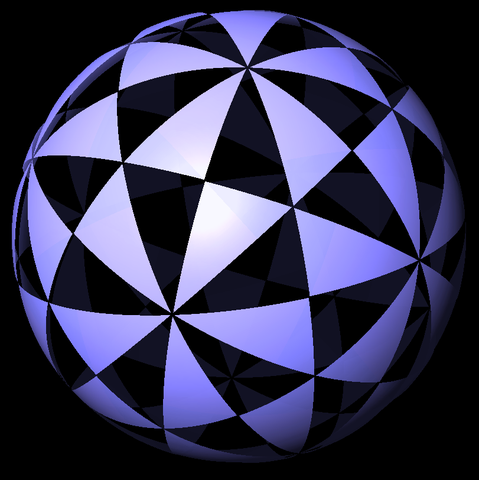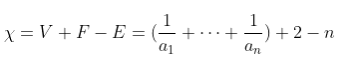# Hurwitz surfaces

The second round of the British Mathematical Olympiad was taken yesterday, with the questions available online from Joseph Myers’ website. Results should be released shortly after marking, which takes place next Saturday.

Recall that a Riemann surface is a complex 1-manifold, or equivalently an orientable real surface locally endowed with the structure of the complex plane, enabling holomorphic functions to be defined on the surface. The most familiar examples are the complex plane itself and its one-point compactification, the Riemann sphere. Another example is an elliptic curve, which is homeomorphic to a torus when viewed as a real 2-manifold.

Now, suppose we have a non-constant holomorphic function f on the sphere. We are interested in the group G of automorphisms φ which preserve the values of f (that is to say, f(φ(z)) = f(z) for all z on the surface); G can be considered to be the symmetry group of f. G must be a finite subgroup of SO(3) and, depending on f, could be either a cyclic group (take$f(z) = z^n$), dihedral group, or one of the polyhedral groups. In the latter case, the largest symmetry group is given by the icosahedral group of order 60:A function with this symmetry is Klein’s icosahedral function, which is involved in a method of solution of the general quintic.

What about symmetry groups of the hyperbolic plane? We desire the one with the most symmetry or, more precisely, the one which minimises the absolute value of the Euler characteristic of the associated orbifold. This can be found by a quick case-bash, since we only care about orientation-preserving symmetries (rotations and translations) so can ignore the more complicated reflections and glide-reflections.

Suppose our orbifold has gyration points (points of rotational symmetry) of orders$a_1, a_2, \dots, a_n \geq 2$. Then the orbifold has an Euler characteristic of:For an explanation of the above identity, note that the orbifold is a sphere with n cone-points (where the ith cone point counts as$\dfrac{1}{a_i}$ of a vertex), which we can cyclically connect to form a polygon with n edges, separating the sphere into two hemispherical faces.

To tile the hyperbolic plane, we require the Euler characteristic to be negative, so we’ll need at least three gyration points. If we have four or more gyration points, the best we can manage is an Euler characteristic of -1/6, corresponding to (2,2,2,3). With three gyration points, however, we want to find the smallest positive value of:$1 - \dfrac{1}{a} - \dfrac{1}{b} - \dfrac{1}{c}$

If two of (a,b,c) are equal, we can double the amount of symmetry by applying the rule (a,b,b) → (2,b,2a). Hence, assume without loss of generality that a < b < c. If a is 3 or more, then the best we can manage is the rather pathetic (3,4,5), which is strictly inferior to (2,4,5). Consequently, we can assume a = 2.

Now, either b = 4 (and the best solution is (2,4,5) with an Euler characteristic of -1/20) or b = 3 (and the best solution is (2,3,7) with an Euler characteristic of -1/42). Clearly the latter is preferable, so the (2,3,7) triangle group has the most symmetry amongst all hyperbolic tessellations.Now, quotients of this tiling will yield compact Riemann surfaces (multi-holed tori) with maximal symmetry (order 84(g − 1), where g is the number of holes, or genus), known as Hurwitz surfaces. The simplest of these is Klein’s quartic, a three-holed torus with symmetry group PSL(2,7) of order 168. Next is the Macbeath surface, which has seven holes and the order-504 symmetry group PSL(2,8).

The next possible genus is 14, giving the order-1092 symmetry group PSL(2,13). Interestingly, there are three distinct Hurwitz surfaces with this symmetry group, known as the first Hurwitz triplet. This can be explained by the fact that in the real subfield of the field obtained by adjoining a principal 7th root of unity to the field of rationals, the number 13 is (up to multiplication by units) a product of three prime factors.

Considering surfaces of a much higher genus, R. A. Wilson demonstrated that the Monster group can be described as the automorphism group of a Hurwitz surface, corresponding to a presentation$1 = g^2 = h^3 = (gh)^7 = \dots$ (with an unknown number of relators omitted). Apart from 64 exceptions (the largest of which is A167), all alternating groups are also Hurwitz.

By solving the equation$|M| = 84(g - 1)$, where |M| is the order of the Monster group, one can deduce that the group acts on an orientable surface with g = 9619255057077534236743570297163223297687552000000001 holes.

This entry was posted in Uncategorized. Bookmark the permalink.

### 5 Responses to Hurwitz surfaces

1.Thomas says:

Is a presentation of the monster group as a hurwitz group known?

2.Unanimous Cowherd says:

“Then the orbifold has an Euler characteristic of:

For an explanation of the above identity, …”

Er, should there be an image or something in between those two lines? I don’t see any.